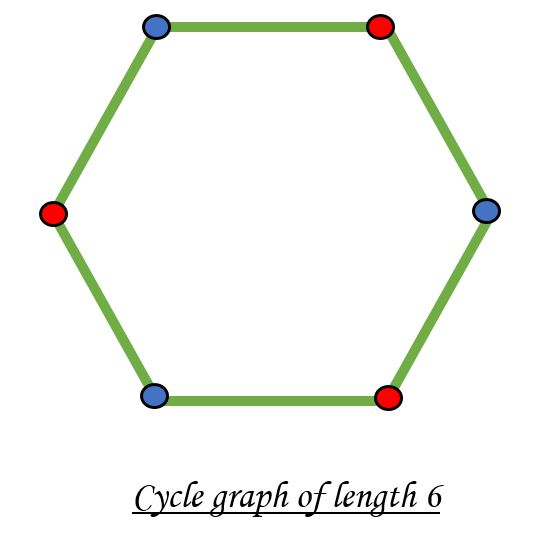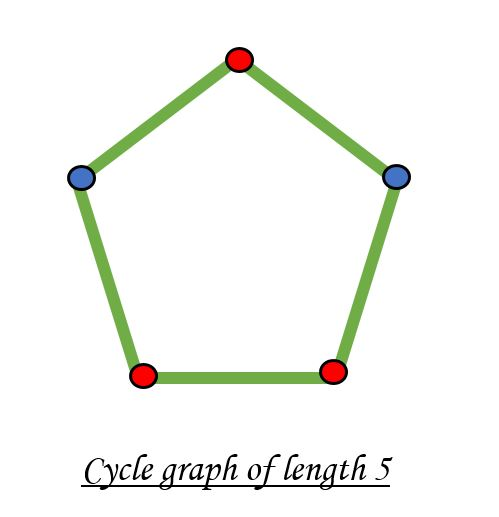# Check if a given graph is Bipartite using DFS

• Difficulty Level : Medium
• Last Updated : 23 Jun, 2022

Given a connected graph, check if the graph is bipartite or not. A bipartite graph is possible if the graph coloring is possible using two colors such that vertices in a set are colored with the same color. Note that it is possible to color a cycle graph with an even cycle using two colors. For example, see the following graph.It is not possible to color a cycle graph with an odd cycle using two colors.In the previous post, an approach using BFS has been discussed. In this post, an approach using DFS has been implemented.

Given below is the algorithm to check for bipartiteness of a graph.

• Use a color[] array which stores 0 or 1 for every node which denotes opposite colors.
• Call the function DFS from any node.
• If the node u has not been visited previously, then assign !color[v] to color[u] and call DFS again to visit nodes connected to u.
• If at any point, color[u] is equal to color[v], then the node is not bipartite.
• Modify the DFS function such that it returns a boolean value at the end.

Below is the implementation of the above approach:

## C++

 `// C++ program to check if a connected``// graph is bipartite or not using DFS``#include ``using` `namespace` `std;` `// function to store the connected nodes``void` `addEdge(vector<``int``> adj[], ``int` `u, ``int` `v)``{``    ``adj[u].push_back(v);``    ``adj[v].push_back(u);``}` `// function to check whether a graph is bipartite or not``bool` `isBipartite(vector<``int``> adj[], ``int` `v,``                 ``vector<``bool``>& visited, vector<``int``>& color)``{` `    ``for` `(``int` `u : adj[v]) {` `        ``// if vertex u is not explored before``        ``if` `(visited[u] == ``false``) {` `            ``// mark present vertic as visited``            ``visited[u] = ``true``;` `            ``// mark its color opposite to its parent``            ``color[u] = !color[v];` `            ``// if the subtree rooted at vertex v is not bipartite``            ``if` `(!isBipartite(adj, u, visited, color))``                ``return` `false``;``        ``}` `        ``// if two adjacent are colored with same color then``        ``// the graph is not bipartite``        ``else` `if` `(color[u] == color[v])``            ``return` `false``;``    ``}``    ``return` `true``;``}` `// Driver Code``int` `main()``{``    ``// no of nodes``    ``int` `N = 6;` `    ``// to maintain the adjacency list of graph``    ``vector<``int``> adj[N + 1];` `    ``// to keep a check on whether``    ``// a node is discovered or not``    ``vector<``bool``> visited(N + 1);` `    ``// to color the vertices``    ``// of graph with 2 color``    ``vector<``int``> color(N + 1);` `    ``// adding edges to the graph``    ``addEdge(adj, 1, 2);``    ``addEdge(adj, 2, 3);``    ``addEdge(adj, 3, 4);``    ``addEdge(adj, 4, 5);``    ``addEdge(adj, 5, 6);``    ``addEdge(adj, 6, 1);` `    ``// marking the source node as visited``    ``visited = ``true``;` `    ``// marking the source node with a color``    ``color = 0;` `    ``// Function to check if the graph``    ``// is Bipartite or not``    ``if` `(isBipartite(adj, 1, visited, color)) {``        ``cout << ``"Graph is Bipartite"``;``    ``}``    ``else` `{``        ``cout << ``"Graph is not Bipartite"``;``    ``}` `    ``return` `0;``}`

## Java

 `// Java program to check if a connected``// graph is bipartite or not using DFS``import` `java.util.*;` `class` `GFG{` `// Function to store the connected nodes``static` `void` `addEdge(ArrayList> adj,``                    ``int` `u, ``int` `v)``{``    ``adj.get(u).add(v);``    ``adj.get(v).add(u);``}` `// Function to check whether a``// graph is bipartite or not``static` `boolean` `isBipartite(ArrayList> adj,``                           ``int` `v, ``boolean` `visited[],``                           ``int` `color[])``{``    ``for``(``int` `u : adj.get(v))``    ``{``        ` `        ``// If vertex u is not explored before``        ``if` `(visited[u] == ``false``)``        ``{``            ` `            ``// Mark present vertic as visited``            ``visited[u] = ``true``;` `            ``// Mark its color opposite to its parent``            ``color[u] = ``1` `- color[v];` `            ``// If the subtree rooted at vertex``            ``// v is not bipartite``            ``if` `(!isBipartite(adj, u, visited, color))``                ``return` `false``;``        ``}` `        ``// If two adjacent are colored with``        ``// same color then the graph is``        ``// not bipartite``        ``else` `if` `(color[u] == color[v])``            ``return` `false``;``    ``}``    ``return` `true``;``}` `// Driver Code``public` `static` `void` `main(String args[])``{``    ` `    ``// No of nodes``    ``int` `N = ``6``;` `    ``// To maintain the adjacency list of graph``    ``ArrayList<``    ``ArrayList> adj = ``new` `ArrayList<``                                  ``ArrayList>(N + ``1``);``    ` `    ``// Initialize all the vertex``    ``for``(``int` `i = ``0``; i <= N; i++)``    ``{``        ``adj.add(``new` `ArrayList());``    ``}``    ` `    ``// To keep a check on whether``    ``// a node is discovered or not``    ``boolean` `visited[] = ``new` `boolean``[N + ``1``];``    ` `    ``// To color the vertices``    ``// of graph with 2 color``    ``int` `color[] = ``new` `int``[N + ``1``];``    ` `    ``// The value '-1' of colorArr[i] is``    ``// used to indicate that no color is``    ``// assigned to vertex 'i'. The value``    ``// 1 is used to indicate first color``    ``// is assigned and value 0 indicates ``    ``// second color is assigned.``    ``Arrays.fill(color, -``1``);` `    ``// Adding edges to the graph``    ``addEdge(adj, ``1``, ``2``);``    ``addEdge(adj, ``2``, ``3``);``    ``addEdge(adj, ``3``, ``4``);``    ``addEdge(adj, ``4``, ``5``);``    ``addEdge(adj, ``5``, ``6``);``    ``addEdge(adj, ``6``, ``1``);` `    ``// Marking the source node as visited``    ``visited[``1``] = ``true``;` `    ``// Marking the source node with a color``    ``color[``1``] = ``0``;` `    ``// Function to check if the graph``    ``// is Bipartite or not``    ``if` `(isBipartite(adj, ``1``, visited, color))``    ``{``        ``System.out.println(``"Graph is Bipartite"``);``    ``}``    ``else``    ``{``        ``System.out.println(``"Graph is not Bipartite"``);``    ``}``}``}` `// This code is contributed by adityapande88`

## Python3

 `# Python3 program to check if a connected``# graph is bipartite or not using DFS`` ` `# Function to store the connected nodes``def` `addEdge(adj, u, v):` `    ``adj[u].append(v)``    ``adj[v].append(u)` `# Function to check whether a graph is``# bipartite or not``def` `isBipartite(adj, v, visited, color):` `    ``for` `u ``in` `adj[v]:`` ` `        ``# If vertex u is not explored before``        ``if` `(visited[u] ``=``=` `False``):`` ` `            ``# Mark present vertic as visited``            ``visited[u] ``=` `True`` ` `            ``# Mark its color opposite to its parent``            ``color[u] ``=` `not` `color[v]`` ` `            ``# If the subtree rooted at vertex v``            ``# is not bipartite``            ``if` `(``not` `isBipartite(adj, u,``                                ``visited, color)):``                ``return` `False``                ` `        ``# If two adjacent are colored with``        ``# same color then the graph is not``        ``# bipartite``        ``elif` `(color[u] ``=``=` `color[v]):``            ``return` `False``    ` `    ``return` `True` `# Driver Code``if` `__name__``=``=``'__main__'``:` `    ``# No of nodes``    ``N ``=` `6`` ` `    ``# To maintain the adjacency list of graph``    ``adj ``=` `[[] ``for` `i ``in` `range``(N ``+` `1``)]`` ` `    ``# To keep a check on whether``    ``# a node is discovered or not``    ``visited ``=` `[``0` `for` `i ``in` `range``(N ``+` `1``)]`` ` `    ``# To color the vertices``    ``# of graph with 2 color``    ``color ``=` `[``0` `for` `i ``in` `range``(N ``+` `1``)]`` ` `    ``# Adding edges to the graph``    ``addEdge(adj, ``1``, ``2``)``    ``addEdge(adj, ``2``, ``3``)``    ``addEdge(adj, ``3``, ``4``)``    ``addEdge(adj, ``4``, ``5``)``    ``addEdge(adj, ``5``, ``6``)``    ``addEdge(adj, ``6``, ``1``)`` ` `    ``# Marking the source node as visited``    ``visited[``1``] ``=` `True`` ` `    ``# Marking the source node with a color``    ``color[``1``] ``=` `0`` ` `    ``# Function to check if the graph``    ``# is Bipartite or not``    ``if` `(isBipartite(adj, ``1``, visited, color)):``        ``print``(``"Graph is Bipartite"``)``    ``else``:``        ``print``(``"Graph is not Bipartite"``)``        ` `# This code is contributed by rutvik_56`

## C#

 `// C# program to check if a connected``// graph is bipartite or not using DFS``using` `System;``using` `System.Collections.Generic;` `class` `GFG{` `// Function to store the connected nodes``static` `void` `addEdge(List> adj,``                    ``int` `u, ``int` `v)``{``    ``adj[u].Add(v);``    ``adj[v].Add(u);``}` `// Function to check whether a``// graph is bipartite or not``static` `bool` `isBipartite(List> adj,``                        ``int` `v, ``bool` `[]visited,``                        ``int` `[]color)``{``    ``foreach``(``int` `u ``in` `adj[v])``    ``{``        ` `        ``// If vertex u is not explored before``        ``if` `(visited[u] == ``false``)``        ``{``            ` `            ``// Mark present vertic as visited``            ``visited[u] = ``true``;` `            ``// Mark its color opposite to its parent``            ``color[u] = 1 - color[v];` `            ``// If the subtree rooted at vertex``            ``// v is not bipartite``            ``if` `(!isBipartite(adj, u, visited, color))``                ``return` `false``;``        ``}``        ` `        ``// If two adjacent are colored with``        ``// same color then the graph is``        ``// not bipartite``        ``else` `if` `(color[u] == color[v])``            ``return` `false``;``    ``}``    ``return` `true``;``}` `// Driver Code``public` `static` `void` `Main(String []args)``{``    ` `    ``// No of nodes``    ``int` `N = 6;` `    ``// To maintain the adjacency list of graph``    ``List> adj = ``new` `List>(N + 1);``    ` `    ``// Initialize all the vertex``    ``for``(``int` `i = 0; i <= N; i++)``    ``{``        ``adj.Add(``new` `List<``int``>());``    ``}``    ` `    ``// To keep a check on whether``    ``// a node is discovered or not``    ``bool` `[]visited = ``new` `bool``[N + 1];``    ` `    ``// To color the vertices``    ``// of graph with 2 color``    ``int` `[]color = ``new` `int``[N + 1];``    ` `    ``// The value '-1' of colorArr[i] is``    ``// used to indicate that no color is``    ``// assigned to vertex 'i'. The value``    ``// 1 is used to indicate first color``    ``// is assigned and value 0 indicates ``    ``// second color is assigned.``    ``for``(``int` `i = 0; i <= N; i++)  ``        ``color[i] = -1;` `    ``// Adding edges to the graph``    ``addEdge(adj, 1, 2);``    ``addEdge(adj, 2, 3);``    ``addEdge(adj, 3, 4);``    ``addEdge(adj, 4, 5);``    ``addEdge(adj, 5, 6);``    ``addEdge(adj, 6, 1);` `    ``// Marking the source node as visited``    ``visited = ``true``;``    ` `    ``// Marking the source node with a color``    ``color = 0;` `    ``// Function to check if the graph``    ``// is Bipartite or not``    ``if` `(isBipartite(adj, 1, visited, color))``    ``{``        ``Console.WriteLine(``"Graph is Bipartite"``);``    ``}``    ``else``    ``{``        ``Console.WriteLine(``"Graph is not Bipartite"``);``    ``}``}``}` `// This code is contributed by Princi Singh`

## Javascript

 ``

Output:

`Graph is Bipartite`

Time Complexity: O(N)
Auxiliary Space: O(N)

My Personal Notes arrow_drop_up